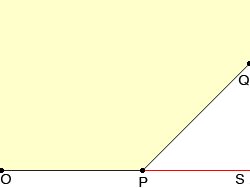Hi my name is anthony, the level is middle, and i am a student. Yes I have another question to help me study for a math exam coming up on january 30. This time the question is about finding interior and/or exterior angles. the question is: In a regular polygon, the ratio of the interior angles to the exterior angles is 3:1. (a) Find the measure of the interior angle. (b) How many sides are there Hi Anthony, I drew three consecutive vertices O, P and Q of the polygon and extended the line OP to a point S.The interior angle OPQ is 3 times as large as the external angle QPS and the sum of these two angles is 180o. Hence 4 time the angle QPS is 180o and thus angle QPS is 45o. Now can you tell how many sides there are? Penny